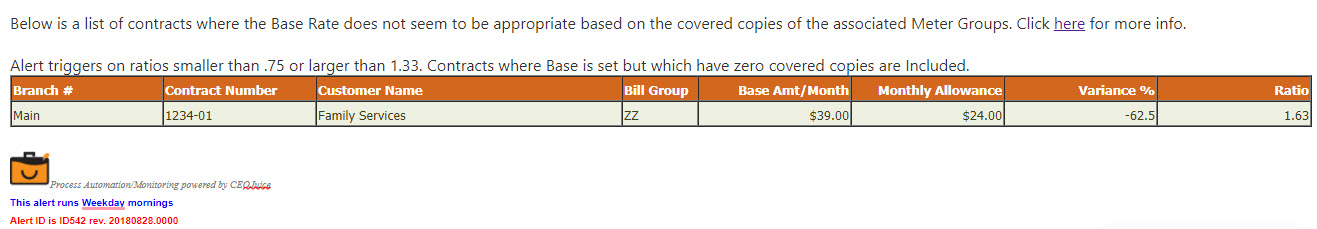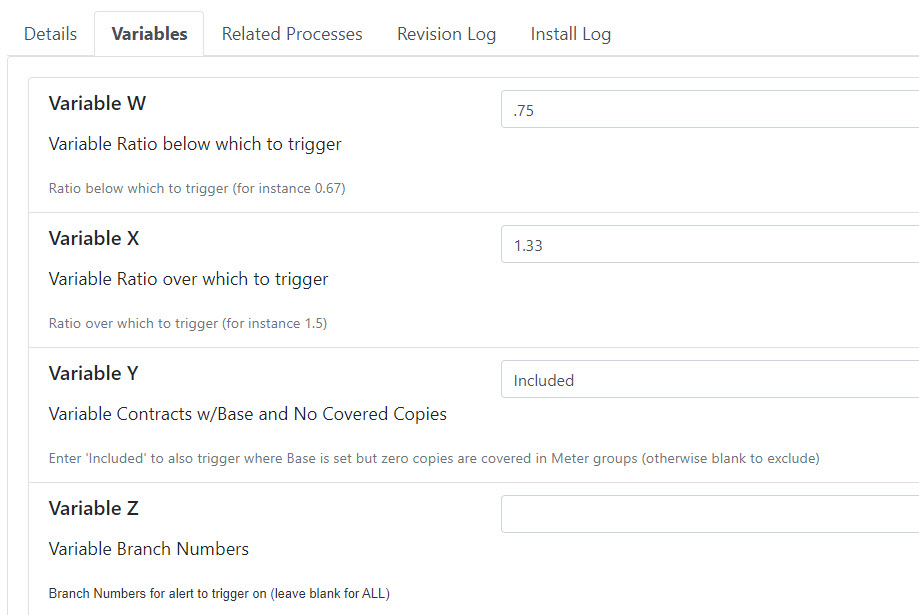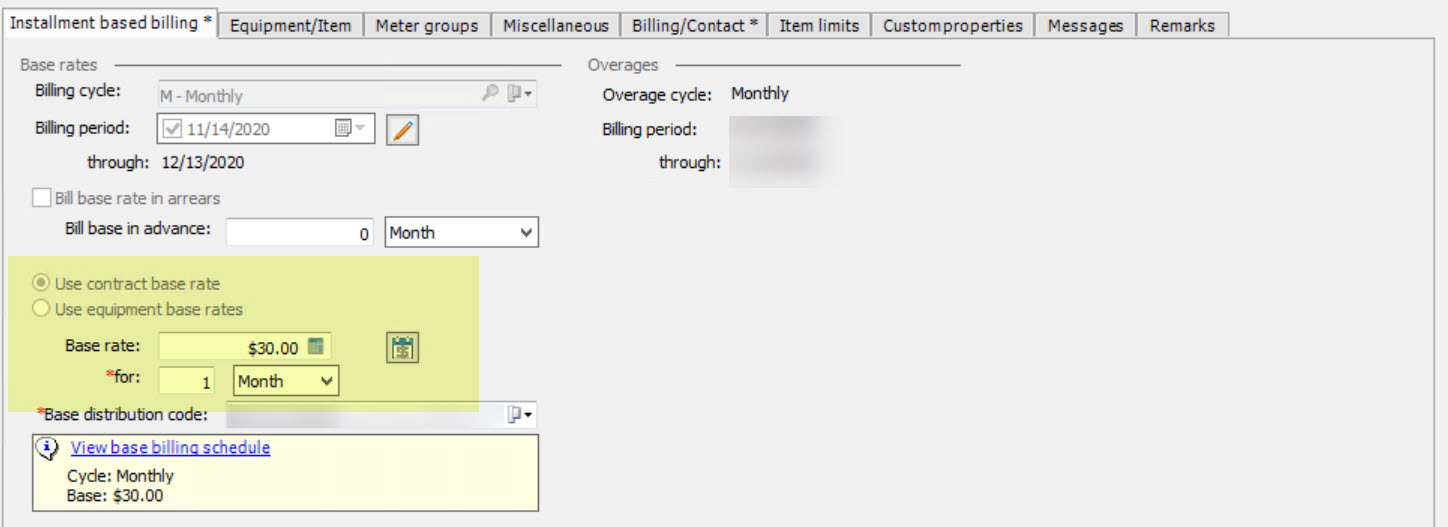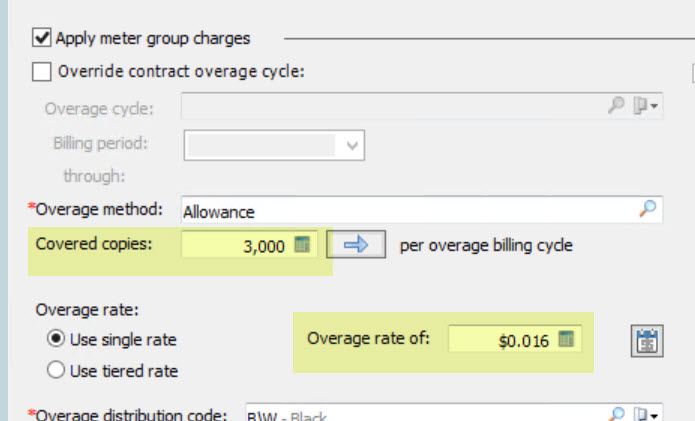Follow

# ID542 - Validate Contract Base Rates against Meter Group Coverage - Overview & Sample

This alert will compare the ratio of Contract Base Rate to the sum of all Meter Group coverages (coverage * overage amount/click) and alert you if the ratio is less than Variable W or higher than Variable X.

Click to Subscribe

#### Overview

Overview

Ever lost money because someone changed a Contract Billing Cycle from Monthly to Quarterly without also changing the Contract Base Rate to match? This alert compares the base rate on the contract to the sum of all the clicks included in the meter groups and reports contracts that are either above or below a set ratio.

There must be some direct relationship between the Meter Group coverage and the Base rate, but if there is then this alert should advise you when someone changes the Billing Cycle incorrectly or if the contract is set up incorrectly in the first place.

Note: This alert does not support tiered base rates (cost-per-click for first X # of pages is different from cost-per-click of next Y # of pages, etc)

Note: If you want the ratios to be reciprocal (to trigger on the same variance in either direction), make sure you do the math correctly. 0.67 = 2/3, the reciprocal is 3/2 = 1.50.

Run Schedule: Weekday mornings

Type of Output: Email

*  *  *

#### Sample

Sample*  *  *

#### Variables

VariablesVariable W: Ratio below which to trigger: Enter the ratio below which the alert will trigger, the default value is 0.75 (If Variable W is set at 0.75 and the base amount billing on the contract is \$200 and the monthly allowance is \$300, this is a ratio of 0.67 and so would trigger the alert as it is under 0.75)

Variable X: Ratio over which to trigger: Enter the ratio over which to trigger the alert, the default is 1.33 (If Variable X is set at 1.33 and the base billing on the contract is \$300 and the monthly allowance is \$200, this is a ratio of 1.5 and so would trigger the alert as it is over 1.33)

Variable Y: Contracts w/Base and No Covered Copies: Enter included to include contracts where there is a base amount set but no covered copies included. Leave blank to exclude these contracts.

Variable Z: Branch Numbers: Enter the branch numbers for the alert to trigger on. Leave blank for all branches.

*  *  *

The Base Amt/Month is the monthly base charge you have set on the contract:The Monthly Allowance is covered copies * overage rate on meter group:The variance is the percentage amount that the customer is potentially being over or undercharged (The variance amount on the alert is calculated as: (Monthly Allowance - Base Amount) / Monthly Allowance).  The ratio is the comparison of the base to the monthly allowance (The ratio amount on the alert is calculated as: Base Amount / Monthly Allowance).

In this example, the customer is being charged a monthly base rate of \$30.00. The monthly allowance is 3,000 (covered copies) * \$0.016 (overage rate) = \$48.00.

The variance on the example contract is (48 - 30)/48 = 0.6 = 60%, indicating the customer is being charged 60% less than they 'should' be.

The ratio on the example contract is 30/48 = 0.625, indicating that the customer is being undercharged. If the alert was set with the default value of 0.75 in Variable W, this contract would trigger the alert.

After a contract triggers it will not show again unless rates are changed and they still fall within the parameters you have set with your variables.  The theory is that we want to let you know that if a competitor walks into your customer they may have an opportunity to offer them lower pricing but once we alert you about that we assume you either made the appropriate changes or that you want the contract to remain as-is and therefore we won't keep bugging you about it.

*  *  *

#### Best Practices & Tips

Best Practices & Tips

Ideally, the variance percent is close to 0%, indicating that the base amount and monthly allowance are roughly in line with each other. A positive variance occurs when the monthly allowance is higher than the base rate; the customer is possibly being undercharged. A negative variance occurs when the monthly allowance is less than the base rate; the customer is possibly being overcharged.

The ratio amount is another way of looking at the over or undercharged amounts. Ideally, the ratio amount is close to 1, indicating that the base rate and monthly allowance are in line with each other. A ratio above 1 indicates that the customer is being overcharged (Base rate is greater than the monthly allowance). A ratio below 1 indicates that the customer is being undercharged (Base rate is less than the monthly allowance).

*  *  *

None at this time

*  *  *